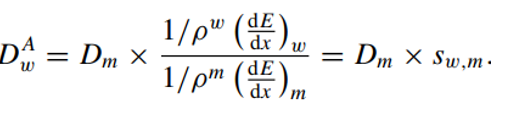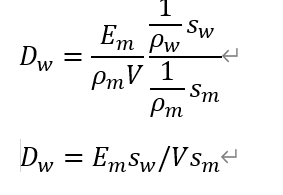# Questions on Dose-H20

Dear experts,
I have found an explanation on how to convert the dose from medium to dose to water. However, I have a few questions that I’m uncertain about:

1. Does the method for calculating the dose to water here utilize the unrestricted stopping power and does it not account for corrections due to delta rays and nuclear interactions?2. Am I correct in understanding that the unit of the result is Gy (gray), rather than GeV/g like the dose scorer?

3. Why they use the different unit, and I’m having difficulty comprehending how the dose to water can be obtained simply by weighting the track length with the stopping power in water. I think there may be other parameters involved, as shown in the following picture.Thank you for your expertise and assistance.

Hello Qinquin

the first equation you quote is the product of a dose to a medium D_m with the dimensionless ratio of stopping powers and densities in water and medium, respectively. As a result, D_w will have the same unit as D_m, for example J/Kg = Gy or GeV/g, the native output by Fluka.

Can you state where you fond the explanation on how DOSE-H2O is calculated, I think it is a somewhat abbreviated explanation.

Also note that the stopping power formulation is valid for charged particles, not photons or neutrons.

Hello, Otto

Thank you for your explanation. I found the explanation on this topic:

I discovered that the results for the DOSE option need to be multiplied by 1.602176462E-7 to obtain reasonable results compared to the DOSE-H2O option.

I am quite confused about how FLUKA calculates the dose to water.

The coefficent

converts a dose expressed in GeV/g to J/kg = Gy.
see 7.22.87. USRBIN — FLUKA Manual, Note 5

Best regards, Thomas @totto